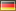## Norm Score Calculator *

The online Norm Score Calculator simplifies the conversion of different types of norm scores (e.g., IQ-scores, T-scores, Standard Scores, Wechsler Subtest Scales and percentiles) into one another. Please fill in a score into the according text field and press return or simply use the slider:

 densitycumulat. z-ScoreT-ScoreIQ-ScoreStand.-S.Wechsler low average high z-Score T-Score IQ-Score Standard-Score WechslerSubscale Percentile Verbal Interpretation: Confidence Interval around the estimated true score:** Reliability: Confidence Level: 95%90%80% 68% Interval: Please have a look at further calculators for confidence intervals and discrepancy analysis

*The Norm Score Calculator uses Chart.js.

#### Statistical Interpretation of Norm Scores

Normal scores help to compare attributes of individuals to a representative population and to determine if the scores lie within the normal range or if they are exceptional. The conversion of individual test results into normal scores is usually based on the assumption that personal traits or abilities are normally distributed within the population. This means that many people have scores around the arithmetic mean and only few people have scores deviating extremely from the population mean. Normal distributions are characterized by their mean M and their standard deviation SD. The standard deviation is a measure for the variability within the distribution (i.e. its width). A normal score is an individual's distance from the population mean quantified in terms of standard deviations.

There are a number of different norm scales. They all more or less yield the same information and can directly be transformed into one another. Probably the most well known scale is the IQ scale with a mean of 100 and a standard deviation of 15. A score of 100 means that the result is completely on average. 115 and more is usually referred to as above average, under 85 is below average.

The Norm Score Calculator also offers the transformation into z-scores (M = 0, SD = 1), T-scores (M = 50, SD = 10), Standard Values (SW score; a norm scale used in German speaking countries; M = 100, SD = 10), and Wechsler Subscale Scores (M = 10, SD = 3).

Percentiles, on the other hand, provide a different kind of information. They specify, which percentage of the reference population scores equal or worse. A percentile of 50 means that 50% of the reference population score equal or worse. A person with percentile 50 therefore shows an average result. Higher scores indicate a better result, lower scores a worse one.